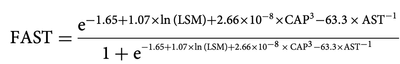## Exponential code

Hi,

Anyone can help me of how to code the below equation into SAS:another one is (1e-6), how to code it in SAS?

1 ACCEPTED SOLUTION

Accepted Solutions

## Re: Exponential code

Hi @ursula,

You can also use the LOGISTIC function to make the code shorter:

`FAST=logistic(-1.65+...);`
4 REPLIES 4

## Re: Exponential code

EXP function for the e

** is power operator so for 2^3 you can do 2**3

@ursula wrote:

Hi,

Anyone can help me of how to code the below equation into SAS:another one is (1e-6), how to code it in SAS?

## Re: Exponential code

The 1E-6 is easy in SAS: That is acceptable as a fixed value though there might sometimes be some issues related to storage precision. The FORMAT you use to display the value might be of interest.

```data example;
file print;
x=1E-6;
put 'Best5. format' x= best5.;
put '9.7 format '   x= 9.7;
run;```

Since you have the same long exponent in the numerator and denominator I would suggest calculating that as another variable and have much simpler code to type like

FAST = exp(thatvar)/ (1+Exp(thatvar));

## Re: Exponential code

The formula can be calculated in a single step, but as the same complex expression occurs twice, it is faster to calculate it in two steps:

``````FAST=exp(-1.65+1.07*log(LSM)+2.66E-8*CAP**3-63.3/AST);
FAST=FAST/(1+FAST);
``````

- by "faster" I mean both the amount of typing to be done, and the CPU time for the calculation.

## Re: Exponential code

Hi @ursula,

You can also use the LOGISTIC function to make the code shorter:

`FAST=logistic(-1.65+...);`
Discussion stats
• 4 replies
• 302 views
• 11 likes
• 5 in conversation